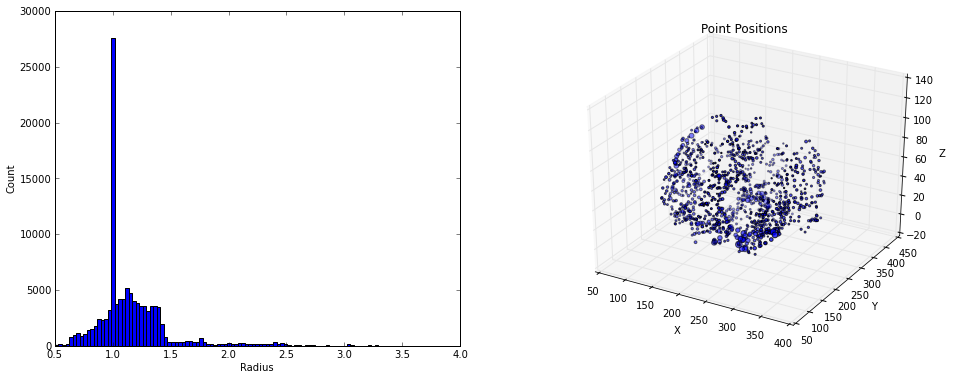This notebook illustrates the TubeTK tube NumPy array data structure and how to create histograms of the properties of a VesselTube.

First, import the function for reading a tube file in as a NumPy array, and read in the file.

In :
import tubetk
from tubetk.numpy import tubes_from_file

import os
filepath = os.path.join(os.path.dirname(tubetk.__file__),
'..', '..', '..',
'MIDAS_Data', 'VascularNetwork.tre')

tubes = tubes_from_file(filepath)

The result is a NumPy Record Array where the fields of the array correspond to the properties of a VesselTubeSpatialObjectPoint.

In :
print(type(tubes))
print(tubes.dtype)
<type 'numpy.ndarray'>
[('ID', '<i4'), ('Position', '<f8', (3,)), ('Color', '<f4', (4,)), ('Tangent', '<f8', (3,)), ('Normal1', '<f8', (3,)), ('Normal2', '<f8', (3,)), ('Radius', '<f4'), ('Alpha1', '<f4'), ('Alpha2', '<f4'), ('Alpha3', '<f4'), ('Medialness', '<f4'), ('Ridgeness', '<f4'), ('Branchness', '<f4'), ('Mark', '|b1')]

The length of the array corresponds to the number of points that make up the tubes.

In :
print(len(tubes))
print(tubes.shape)
110655
(110655,)

Individual points can be sliced, or views can be created on individual fields.

In :
print('Entire points 0, 2:')
print(tubes[:4:2])

print('\nPosition of points 0, 2')
print(tubes['Position'][:4:2])
Entire points 0, 2:
[ (-1, [249.4600067138672, 185.70599365234375, 0.5305629968643188], [1.0, 0.0, 0.0, 1.0], [0.16296979670004633, -0.9853404631248955, -0.050448162428001125], [0.9853404631248955, 0.16296979670004633, 0.0], [0.008221526774782259, -0.04970861573060659, 0.997454982907638], 2.4312500953674316, 0.0, 0.0, 0.0, 0.0, 0.0, 0.0, False)
(-1, [249.46099853515625, 185.6999969482422, 0.5302559733390808], [1.0, 0.0, 0.0, 1.0], [0.26352887911124834, -0.964488943346244, -0.017708981823207896], [0.964488943346244, 0.26352887911124834, 0.0], [0.004666828130071448, -0.017080117166403626, 0.9996863919627852], 2.4308600425720215, 0.0, 0.0, 0.0, 0.0, 0.0, 0.0, False)]

Position of points 0, 2
[[ 249.46000671  185.70599365    0.530563  ]
[ 249.46099854  185.69999695    0.53025597]]

We can easily create a histogram of the radii or visualize the point positions.

In :
%pylab inline
from mpl_toolkits.mplot3d import Axes3D
import matplotlib.pyplot as plt

fig = plt.figure(figsize=(16, 6))

ax.set_ylabel('Count')

ax = fig.add_subplot(1, 2, 2, projection='3d')
subsample = 100
position = tubes['Position'][::subsample]